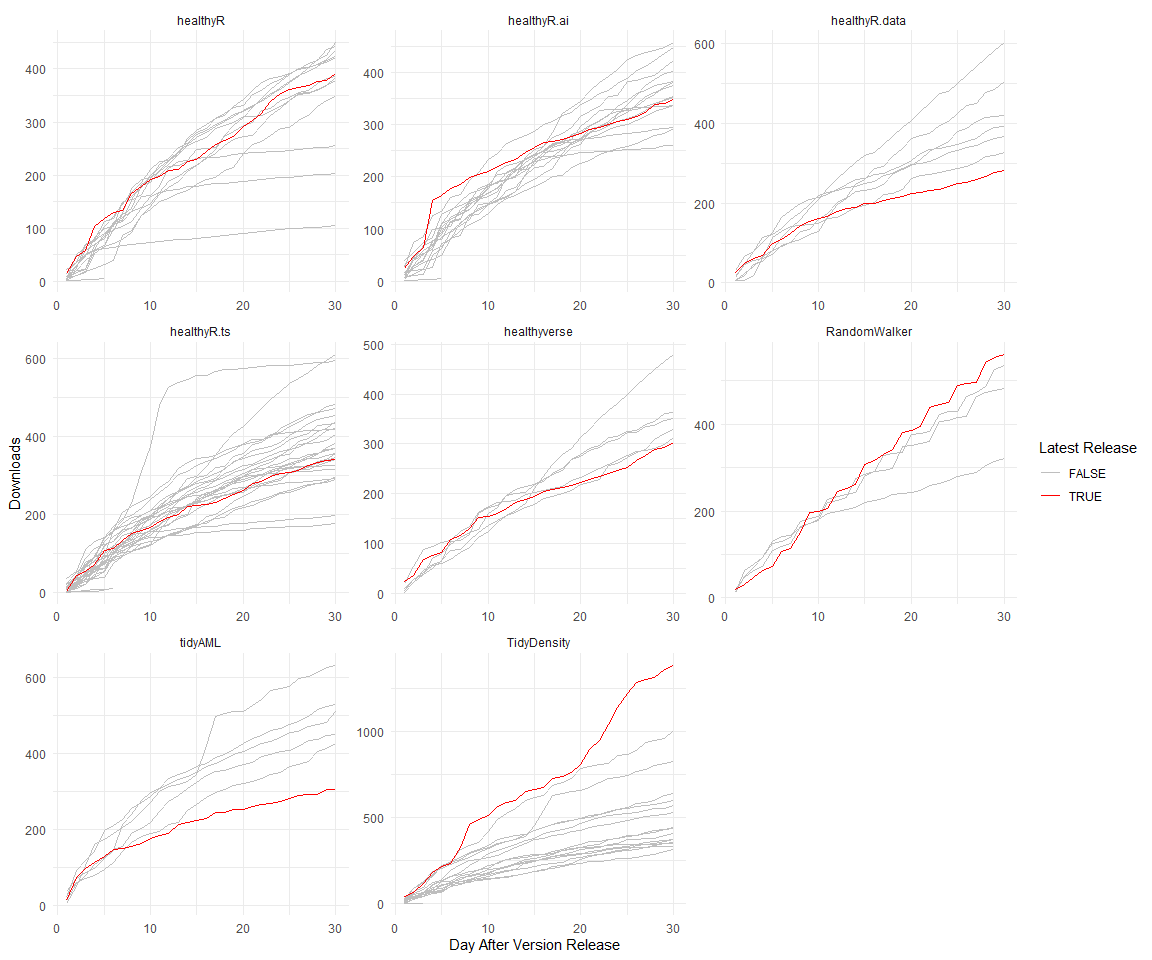Steven P. Sanderson II, MPH - Date: 07 December, 2023

This repo contains the analysis of downloads of my `R` packages:

All of which follow the “analyses as package” philosophy this repo itself is an `R` package that can installed using `remotes::install_github()`.

I have forked this project itself from `ggcharts-analysis`.

While I analyze `healthyverse` packages here, the functions are written in a way that you can analyze any CRAN package with a slight modification to the `download_log` function.

This file was last updated on December 07, 2023.

``````library(packagedownloads)
library(tidyverse)
library(patchwork)
library(timetk)
library(knitr)
library(leaflet)
library(htmltools)
library(tmaptools)
library(mapview)
library(countrycode)
library(htmlwidgets)
library(webshot)
library(rmarkdown)
library(dtplyr)
``````
``````start_date      <- Sys.Date() - 9 #as.Date("2020-11-15")
end_date        <- Sys.Date() - 2
interactive     <- FALSE
pkg_release_date_tbl()
``````

# Last Full Day Data

``````downloads            <- total_downloads |> filter(date == max(date))

f <- function(date) format(date, "%b %d, %Y")
patchwork_theme <- theme_classic(base_size = 24) +
theme(
plot.title   = element_text(face = "bold"),
plot.caption = element_text(size = 14)
)
p1 + p2 +
plot_annotation(
title    = "healthyverse Packages - Last Full Day",
caption  = glue::glue("Source: RStudio CRAN Logs for {f(end_date)}"),
theme    = patchwork_theme
)
``````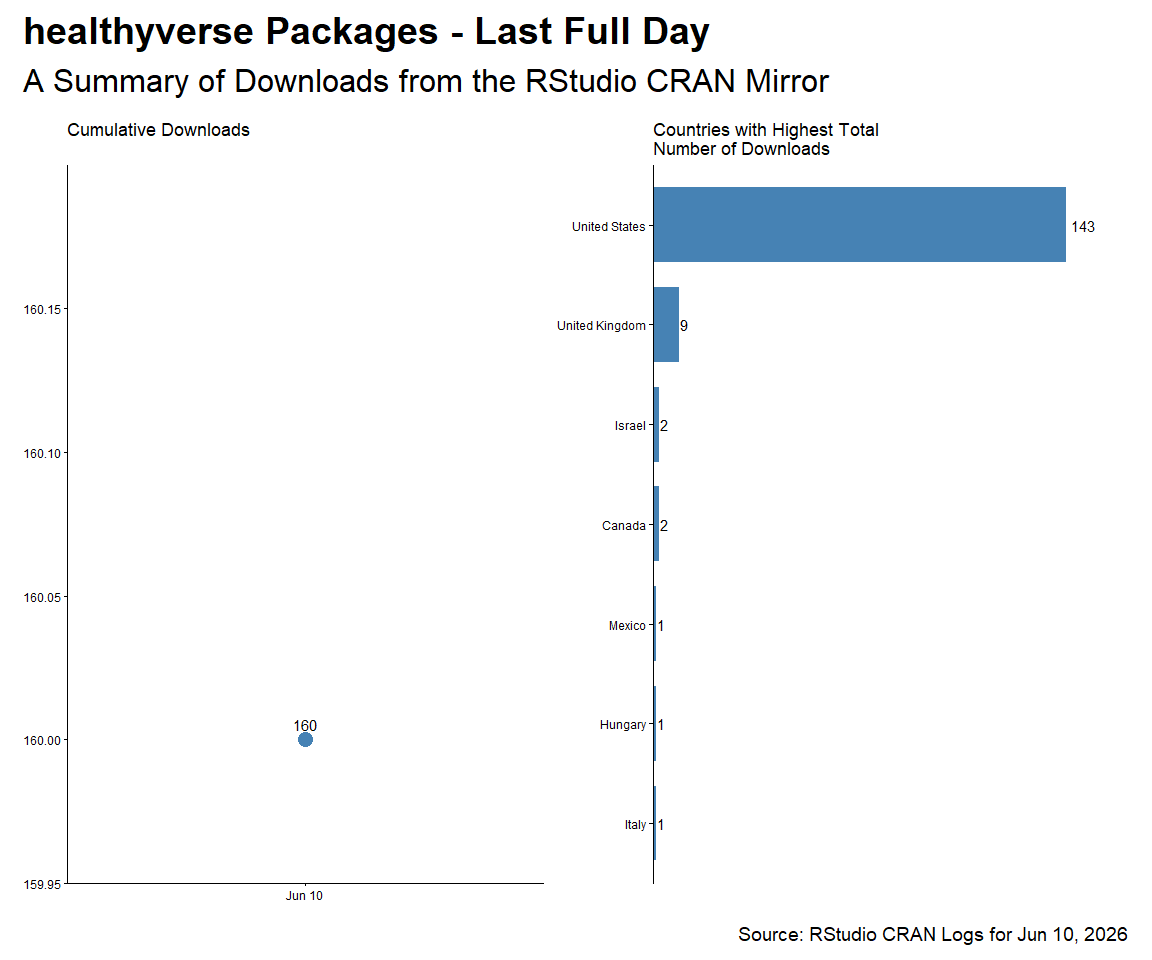``````downloads |>
count(package, version) |>
tidyr::pivot_wider(
id_cols       = version
, names_from  = package
, values_from = n
, values_fill = 0
) |>
arrange(version) |>
kable()
``````
version TidyDensity healthyR healthyR.ai healthyR.data healthyR.ts healthyverse tidyAML
0.0.13 0 0 6 0 0 0 0
0.0.3 0 0 0 0 0 0 9
0.0.6 0 0 1 0 0 0 0
0.1.1 0 0 0 0 1 0 0
0.1.2 0 1 0 0 0 0 0
0.1.8 0 1 0 0 1 0 0
0.2.1 0 6 0 0 0 0 0
0.3.0 0 0 0 0 8 0 0
1.0.0 0 0 0 0 0 1 0
1.0.1 1 0 0 2 0 0 0
1.0.2 0 0 0 0 0 1 0
1.0.3 0 0 0 6 0 0 0
1.0.4 0 0 0 0 0 6 0
1.2.6 16 0 0 0 0 0 0
``````downloads |>
count(package) |>
tidyr::pivot_wider(
names_from = package,
values_from = n,
values_fill = 0
) |>
kable()
``````
TidyDensity healthyR healthyR.ai healthyR.data healthyR.ts healthyverse tidyAML
17 8 7 8 10 8 9

# Current Trend

Here are the current 7 day trends for the `healthyverse` suite of packages.

``````downloads            <- total_downloads[date >= start_date]

f <- function(date) format(date, "%b %d, %Y")
patchwork_theme <- theme_classic(base_size = 24) +
theme(
plot.title   = element_text(face = "bold"),
plot.caption = element_text(size = 14)
)
p1 + p2 + p3 + p4 +
plot_annotation(
title    = "healthyverse Packages - 7 Day Trend",
caption  = glue::glue("Source: RStudio CRAN Logs ({f(start_date)} to {f(end_date)})"),
theme    = patchwork_theme
)
``````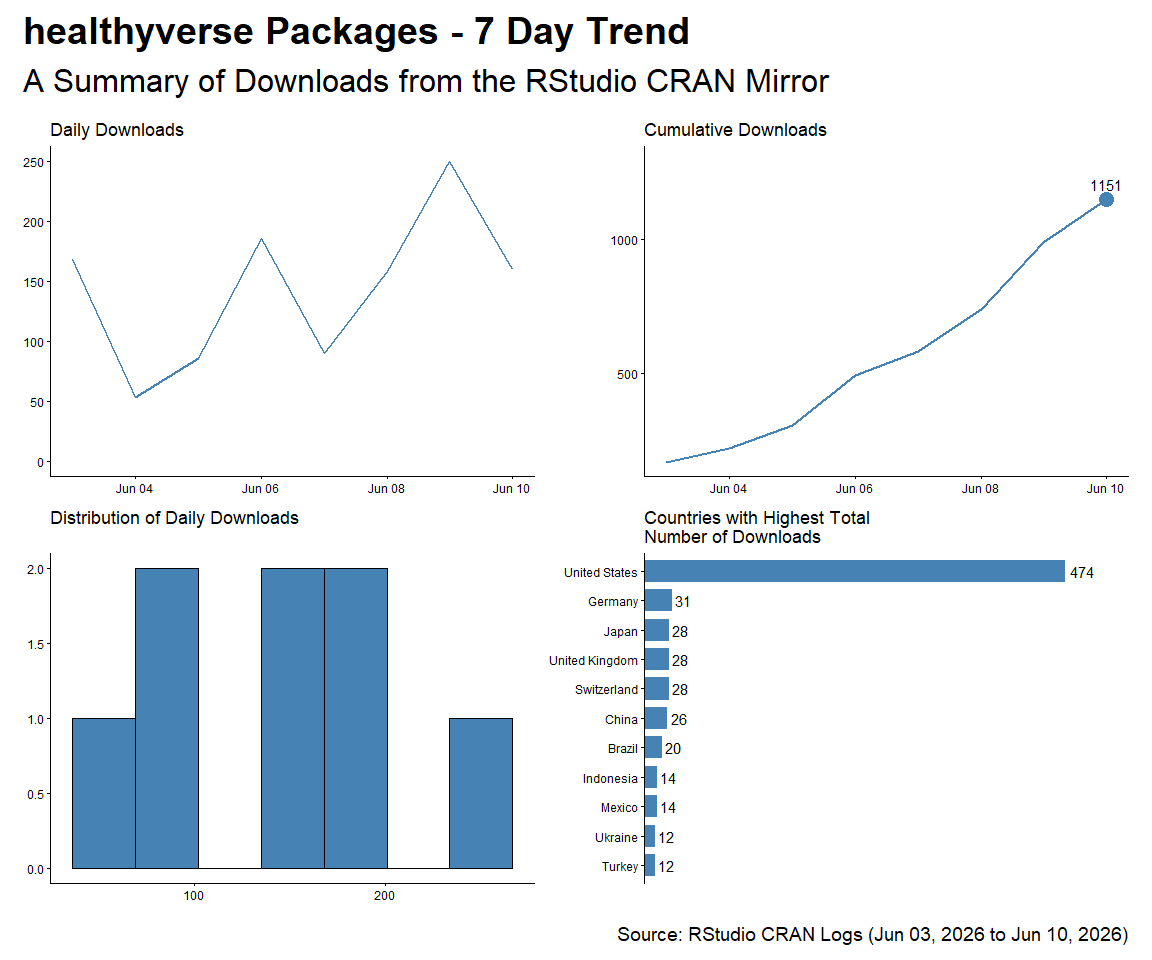# Since Inception

``````start_date <- as.Date("2020-11-15")

f <- function(date) format(date, "%b %d, %Y")
patchwork_theme <- theme_classic(base_size = 24) +
theme(
plot.title   = element_text(face = "bold"),
plot.caption = element_text(size = 14)
)
p1 + p2 + p3 + p4 +
plot_annotation(
title    = "healthyR packages are on the Rise",
subtitle = "A Summary of Downloads from the RStudio CRAN Mirror - Since Inception",
caption  = glue::glue("Source: RStudio CRAN Logs ({f(start_date)} to {f(end_date)})"),
theme    = patchwork_theme
)
``````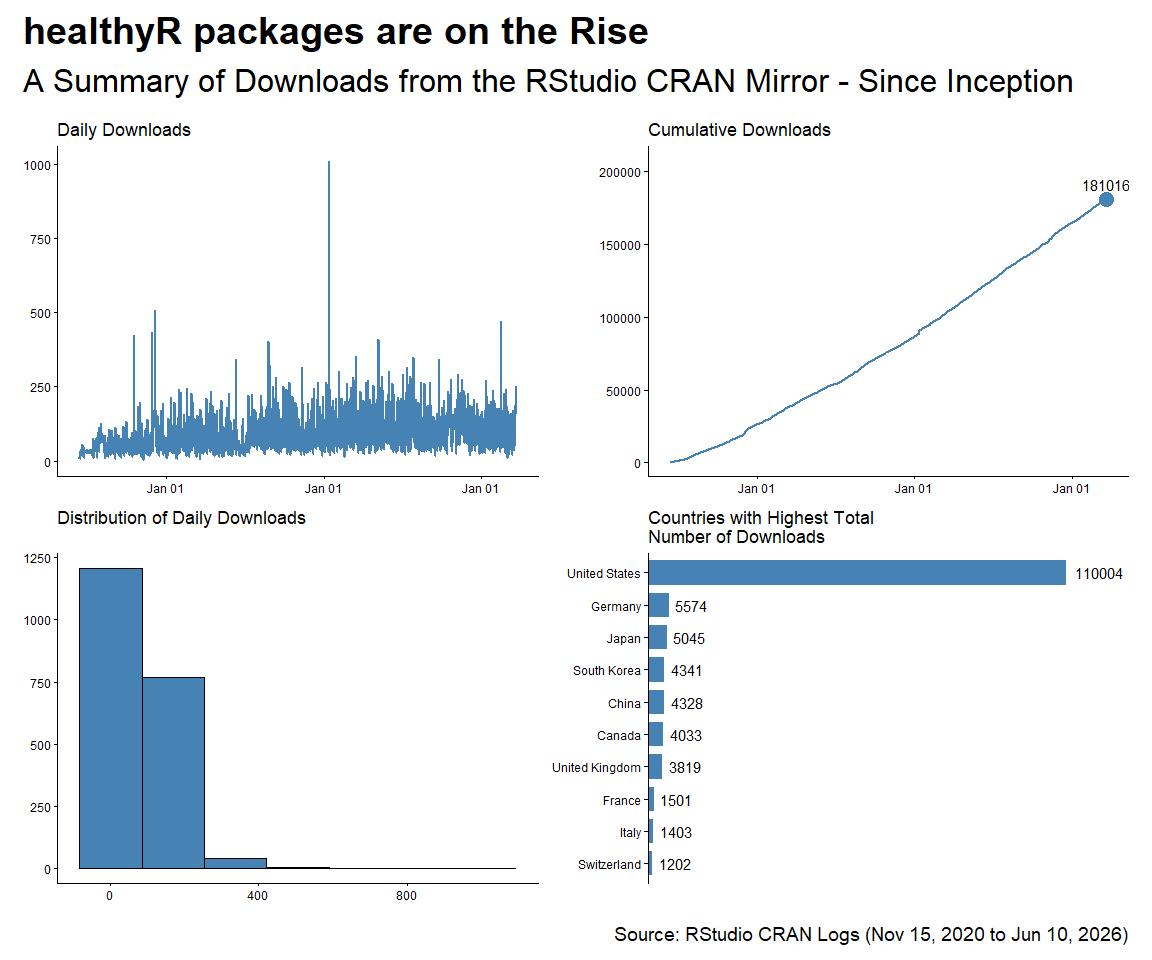# By Release Date

``````pkg_tbl <- readRDS("pkg_release_tbl.rds")
group_by(package) %>%
summarise_by_time(
.date_var = date,
.by = "week",
N = n()
) %>%
ungroup() %>%
select(date, package, N)

dl_tbl %>%
ggplot(aes(date, log1p(N))) +
theme_bw() +
geom_point(aes(group = package, color = package), size = 1) +
geom_line(aes(group = package, color = package)) +
geom_smooth(method = "loess", color = "black",  se = FALSE) +
geom_vline(
data = pkg_tbl
, aes(xintercept = as.numeric(date))
, color = "red"
, lwd = 1
, lty = "solid"
) +
facet_wrap(package ~., ncol = 2, scales = "free_x") +
theme_minimal() +
labs(
subtitle = "Vertical lines represent release dates",
x = "Date",
y = "log1p(Counts)",
color = "Package"
) +
theme(legend.position = "bottom")
``````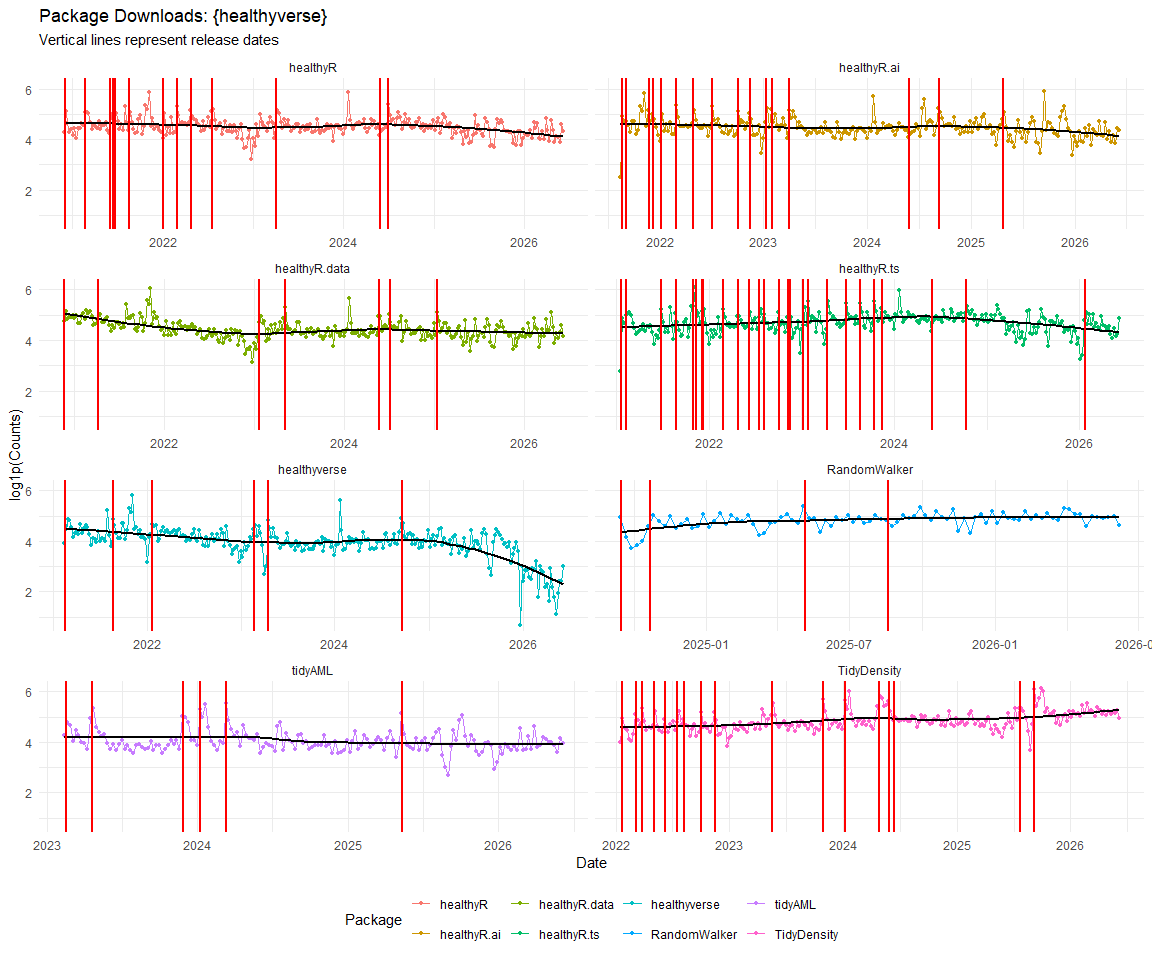``````dl_tbl %>%
select(date, N) %>%
summarise_by_time(
.date_var = date,
.by = "week",
Actual = sum(N, na.rm = TRUE)
) %>%
tk_augment_differences(.value = Actual, .differences = 1) %>%
tk_augment_differences(.value = Actual, .differences = 2) %>%
rename(velocity = contains("_diff1")) %>%
rename(acceleration = contains("_diff2")) %>%
pivot_longer(-date) %>%
mutate(name = str_to_title(name)) %>%
mutate(name = as_factor(name)) %>%
ggplot(aes(x = date, y = log1p(value), group = name)) +
geom_point(alpha = .2) +
geom_vline(
data = pkg_tbl
, aes(xintercept = as.numeric(date), color = package)
, lwd = 1
, lty = "solid"
) +
facet_wrap(name ~ ., ncol = 1, scale = "free") +
theme_minimal() +
labs(
subtitle = "Vertical Lines Indicate a CRAN Release date for a package.",
x = "Date",
y = "",
color = ""
) +
theme(legend.position = "bottom")
``````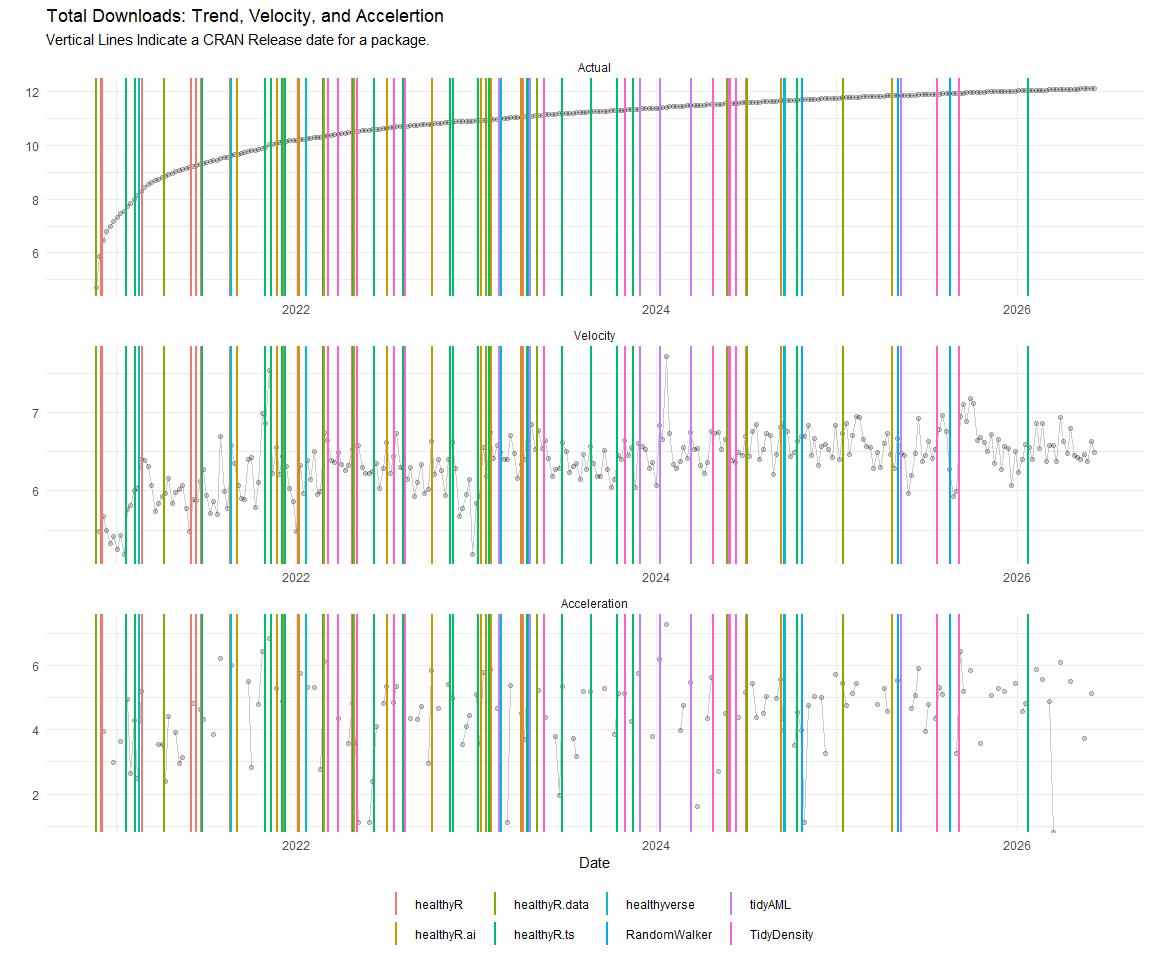A `leaflet` map of countries where a package has been downloaded.

``````mapping_dataset() %>%
knitr::kable()
``````
country latitude longitude display_name icon
United States 39.78373 -100.445882 United States https://nominatim.openstreetmap.org/ui/mapicons/poi_boundary_administrative.p.20.png
United Kingdom 54.70235 -3.276575 United Kingdom https://nominatim.openstreetmap.org/ui/mapicons/poi_boundary_administrative.p.20.png
Hong Kong SAR China 22.35063 114.184916 香港 Hong Kong, 中国 https://nominatim.openstreetmap.org/ui/mapicons/poi_boundary_administrative.p.20.png
``````l <- map_leaflet()
cliprect = "viewport")
``````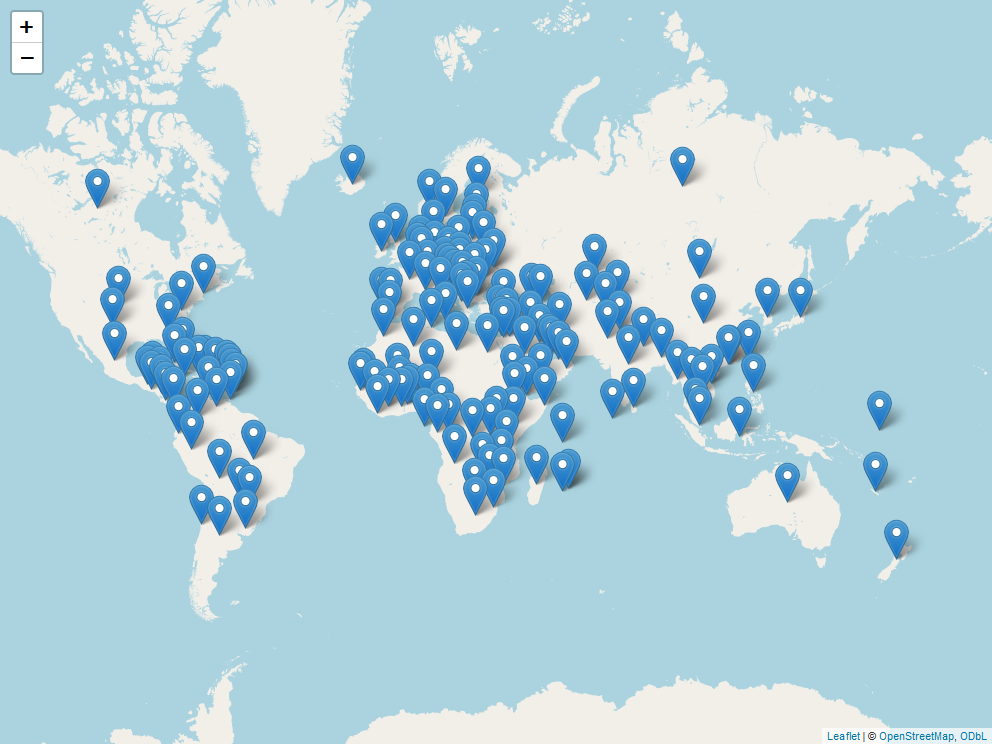To date there has been downloads in a total of 144 different countries.

# Analysis by Package

## healthyR

``````start_date <- as.Date("2020-11-15")
pkg <- "healthyR"

, pkg = pkg)
, pkg = pkg)

f <- function(date) format(date, "%b %d, %Y")
patchwork_theme <- theme_classic(base_size = 24) +
theme(
plot.title   = element_text(face = "bold"),
plot.caption = element_text(size = 14)
)
p1 + p2 + p3 + p4 +
plot_annotation(
title    = glue::glue("Package: {pkg}"),
subtitle = "A Summary of Downloads from the RStudio CRAN Mirror - Since Inception",
caption  = glue::glue("Source: RStudio CRAN Logs ({f(start_date)} to {f(end_date)})"),
theme    = patchwork_theme
)
``````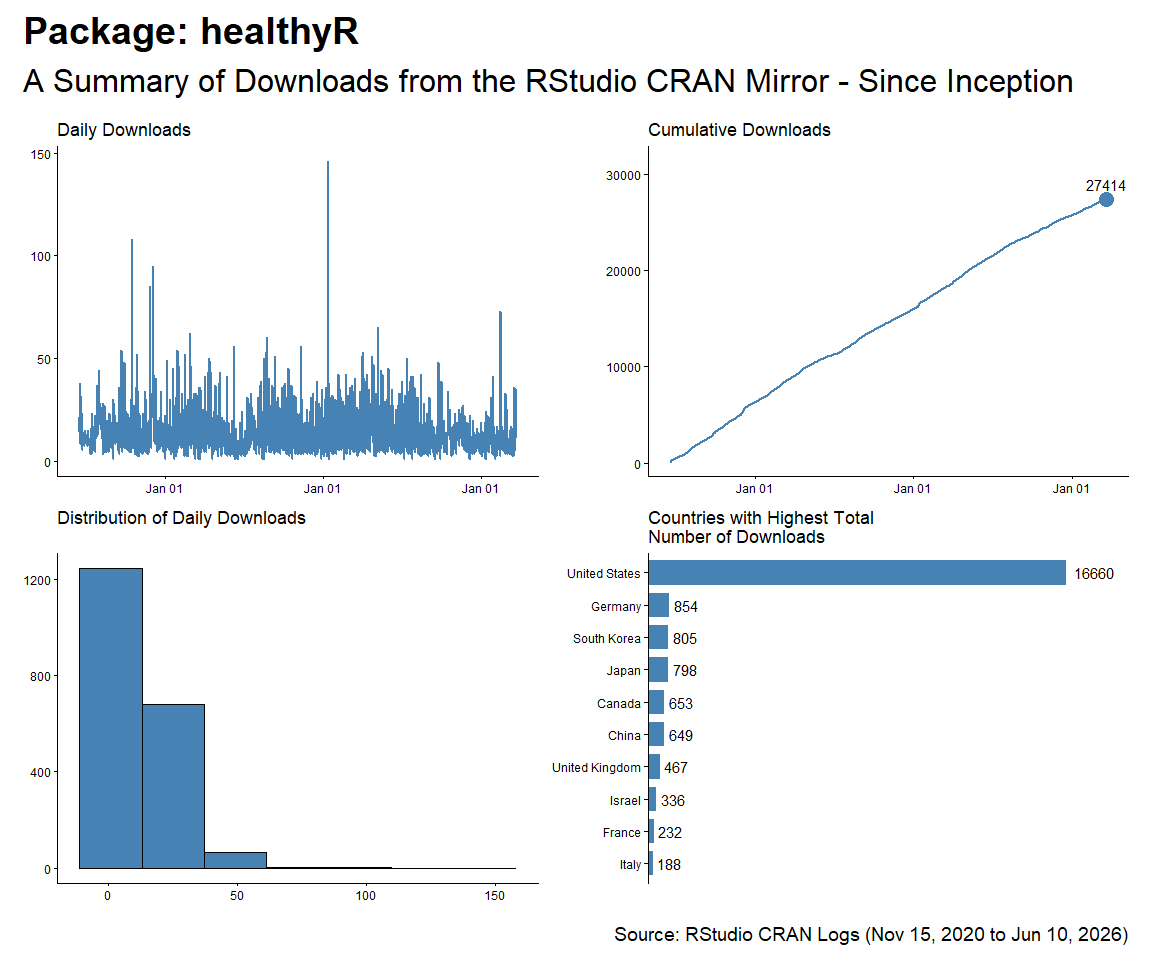## healthyR.ts

``````start_date <- as.Date("2020-11-15")
pkg <- "healthyR.ts"

, pkg = pkg)
, pkg = pkg)

f <- function(date) format(date, "%b %d, %Y")
patchwork_theme <- theme_classic(base_size = 24) +
theme(
plot.title   = element_text(face = "bold"),
plot.caption = element_text(size = 14)
)
p1 + p2 + p3 + p4 +
plot_annotation(
title    = glue::glue("Package: {pkg}"),
subtitle = "A Summary of Downloads from the RStudio CRAN Mirror - Since Inception",
caption  = glue::glue("Source: RStudio CRAN Logs ({f(start_date)} to {f(end_date)})"),
theme    = patchwork_theme
)
``````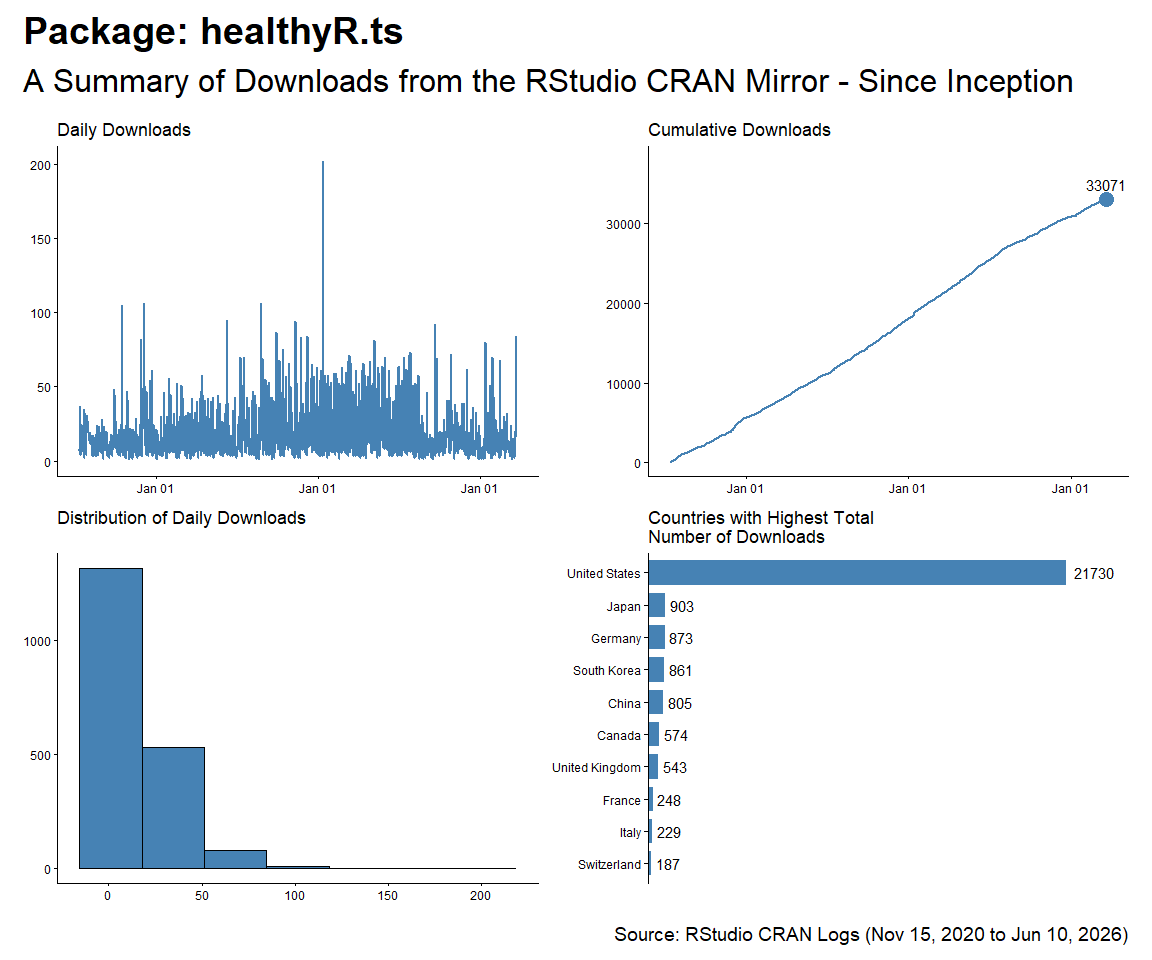## healthyR.data

``````start_date <- as.Date("2020-11-15")
pkg <- "healthyR.data"

, pkg = pkg)
, pkg = pkg)

f <- function(date) format(date, "%b %d, %Y")
patchwork_theme <- theme_classic(base_size = 24) +
theme(
plot.title   = element_text(face = "bold"),
plot.caption = element_text(size = 14)
)
p1 + p2 + p3 + p4 +
plot_annotation(
title    = glue::glue("Package: {pkg}"),
subtitle = "A Summary of Downloads from the RStudio CRAN Mirror - Since Inception",
caption  = glue::glue("Source: RStudio CRAN Logs ({f(start_date)} to {f(end_date)})"),
theme    = patchwork_theme
)
``````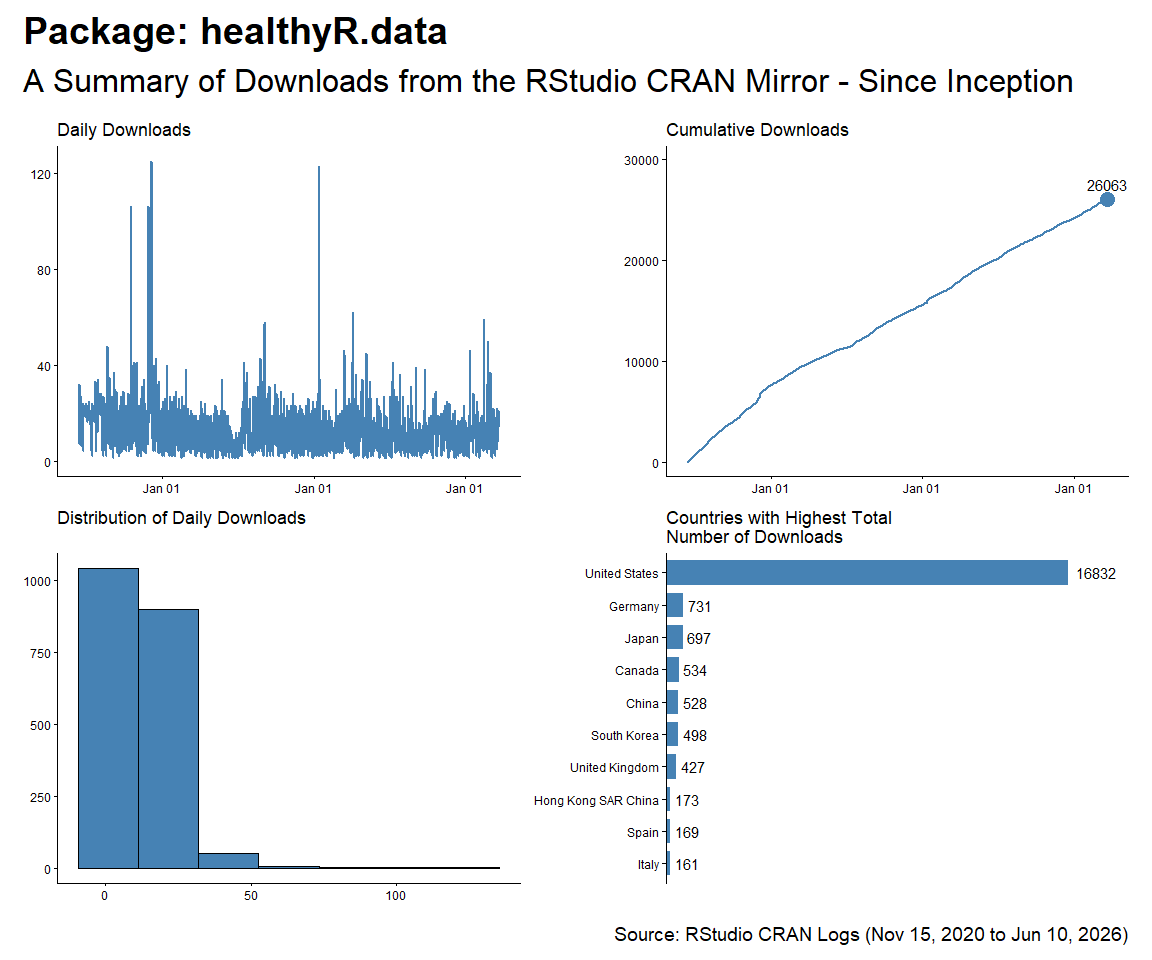## healthyR.ai

``````start_date <- as.Date("2020-11-15")
pkg <- "healthyR.ai"

, pkg = pkg)
, pkg = pkg)

f <- function(date) format(date, "%b %d, %Y")
patchwork_theme <- theme_classic(base_size = 24) +
theme(
plot.title   = element_text(face = "bold"),
plot.caption = element_text(size = 14)
)
p1 + p2 + p3 + p4 +
plot_annotation(
title    = glue::glue("Package: {pkg}"),
subtitle = "A Summary of Downloads from the RStudio CRAN Mirror - Since Inception",
caption  = glue::glue("Source: RStudio CRAN Logs ({f(start_date)} to {f(end_date)})"),
theme    = patchwork_theme
)
``````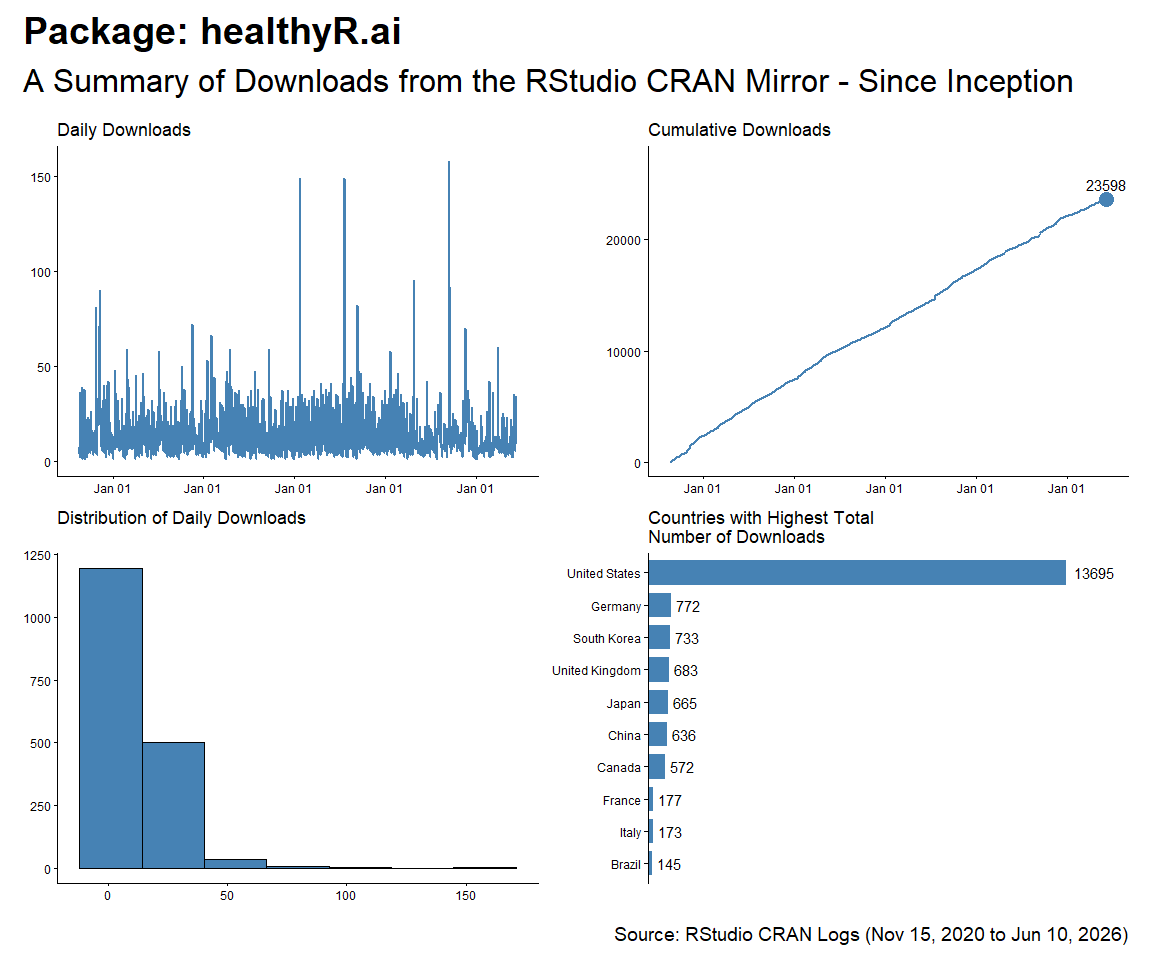## healthyverse

``````start_date <- as.Date("2020-11-15")
pkg <- "healthyverse"

, pkg = pkg)
, pkg = pkg)

f <- function(date) format(date, "%b %d, %Y")
patchwork_theme <- theme_classic(base_size = 24) +
theme(
plot.title   = element_text(face = "bold"),
plot.caption = element_text(size = 14)
)
p1 + p2 + p3 + p4 +
plot_annotation(
title    = glue::glue("Package: {pkg}"),
subtitle = "A Summary of Downloads from the RStudio CRAN Mirror - Since Inception",
caption  = glue::glue("Source: RStudio CRAN Logs ({f(start_date)} to {f(end_date)})"),
theme    = patchwork_theme
)
``````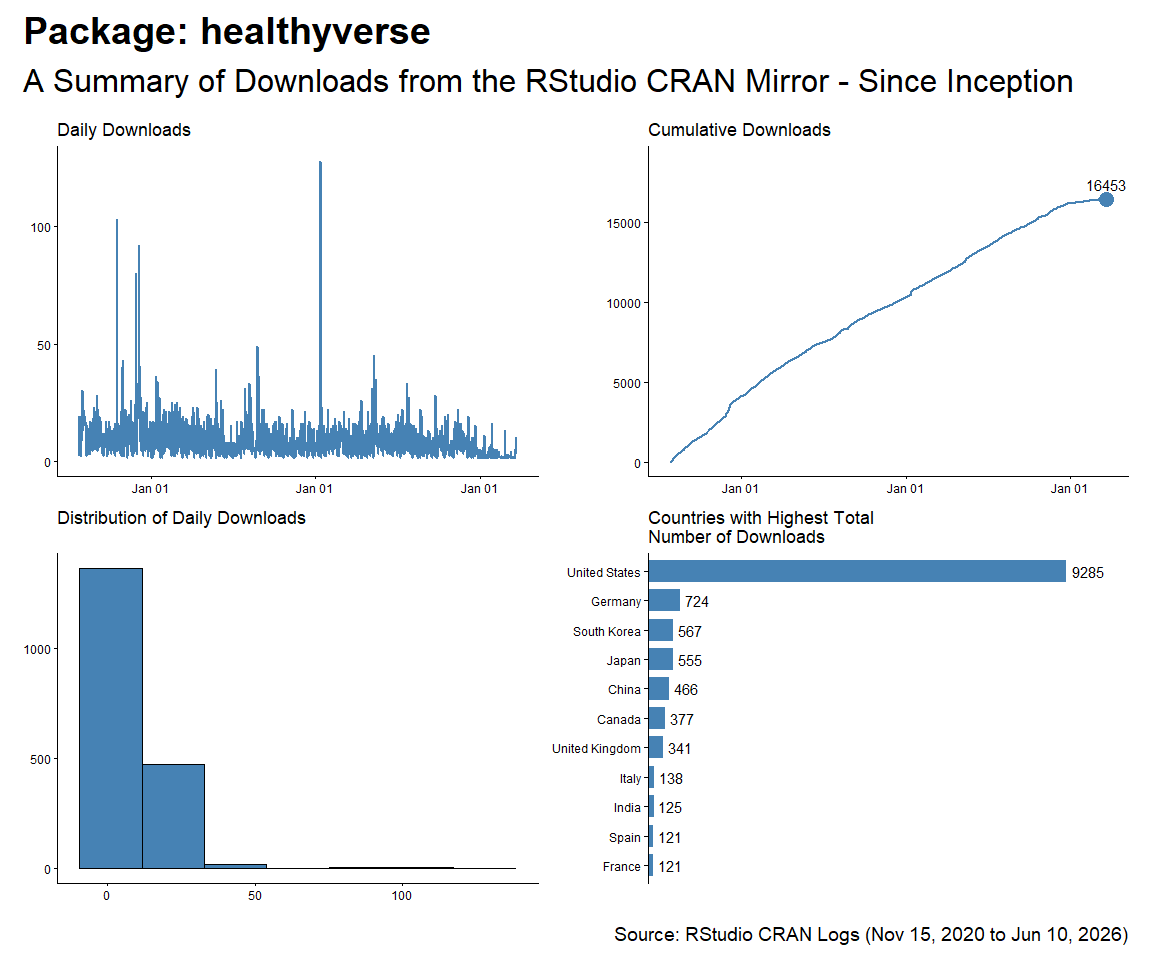## TidyDensity

``````start_date <- as.Date("2020-11-15")
pkg <- "TidyDensity"

, pkg = pkg)
, pkg = pkg)

f <- function(date) format(date, "%b %d, %Y")
patchwork_theme <- theme_classic(base_size = 24) +
theme(
plot.title   = element_text(face = "bold"),
plot.caption = element_text(size = 14)
)
p1 + p2 + p3 + p4 +
plot_annotation(
title    = glue::glue("Package: {pkg}"),
subtitle = "A Summary of Downloads from the RStudio CRAN Mirror - Since Inception",
caption  = glue::glue("Source: RStudio CRAN Logs ({f(start_date)} to {f(end_date)})"),
theme    = patchwork_theme
)
``````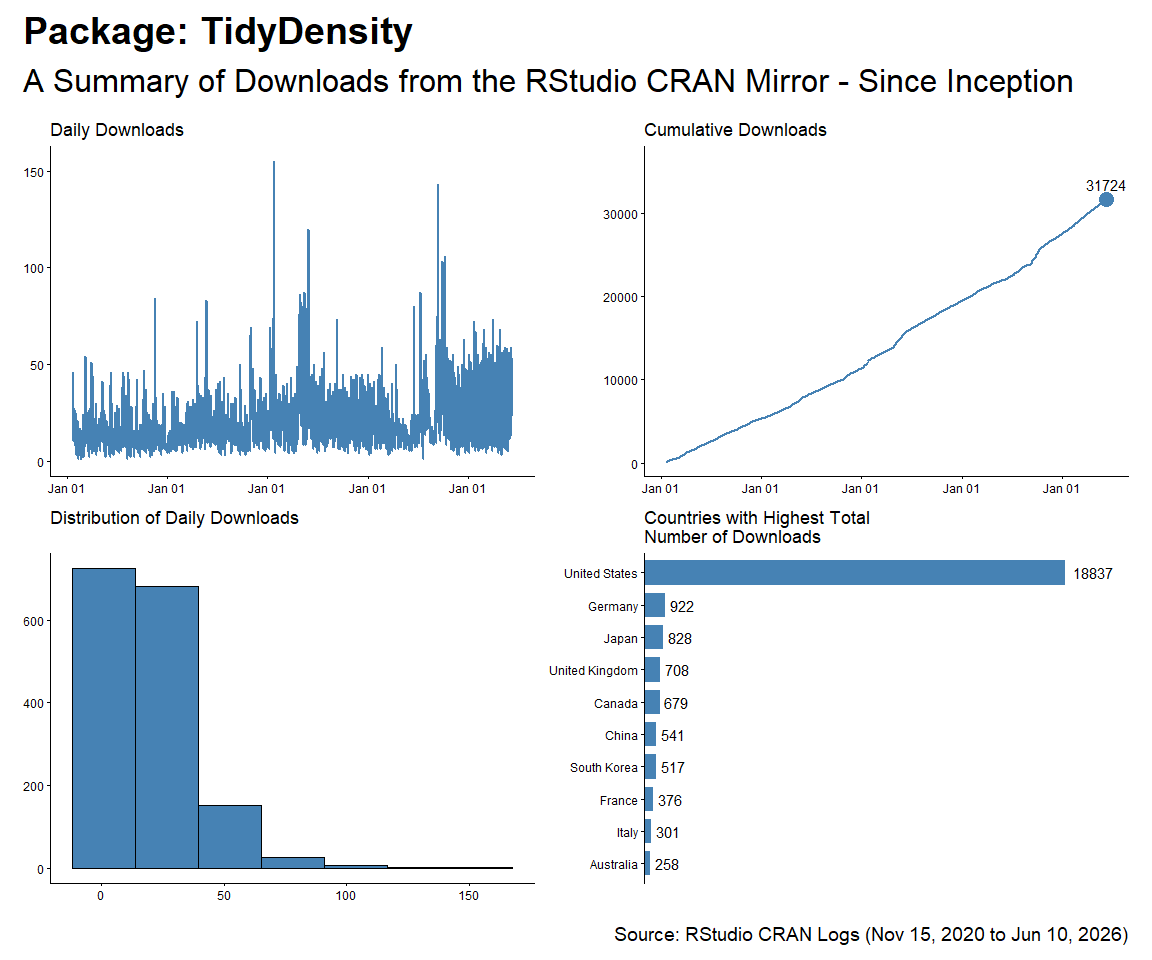## tidyAML

``````start_date <- as.Date("2023-02-13")
pkg <- "tidyAML"

, pkg = pkg)
, pkg = pkg)

f <- function(date) format(date, "%b %d, %Y")
patchwork_theme <- theme_classic(base_size = 24) +
theme(
plot.title   = element_text(face = "bold"),
plot.caption = element_text(size = 14)
)
p1 + p2 + p3 + p4 +
plot_annotation(
title    = glue::glue("Package: {pkg}"),
subtitle = "A Summary of Downloads from the RStudio CRAN Mirror - Since Inception",
caption  = glue::glue("Source: RStudio CRAN Logs ({f(start_date)} to {f(end_date)})"),
theme    = patchwork_theme
)
``````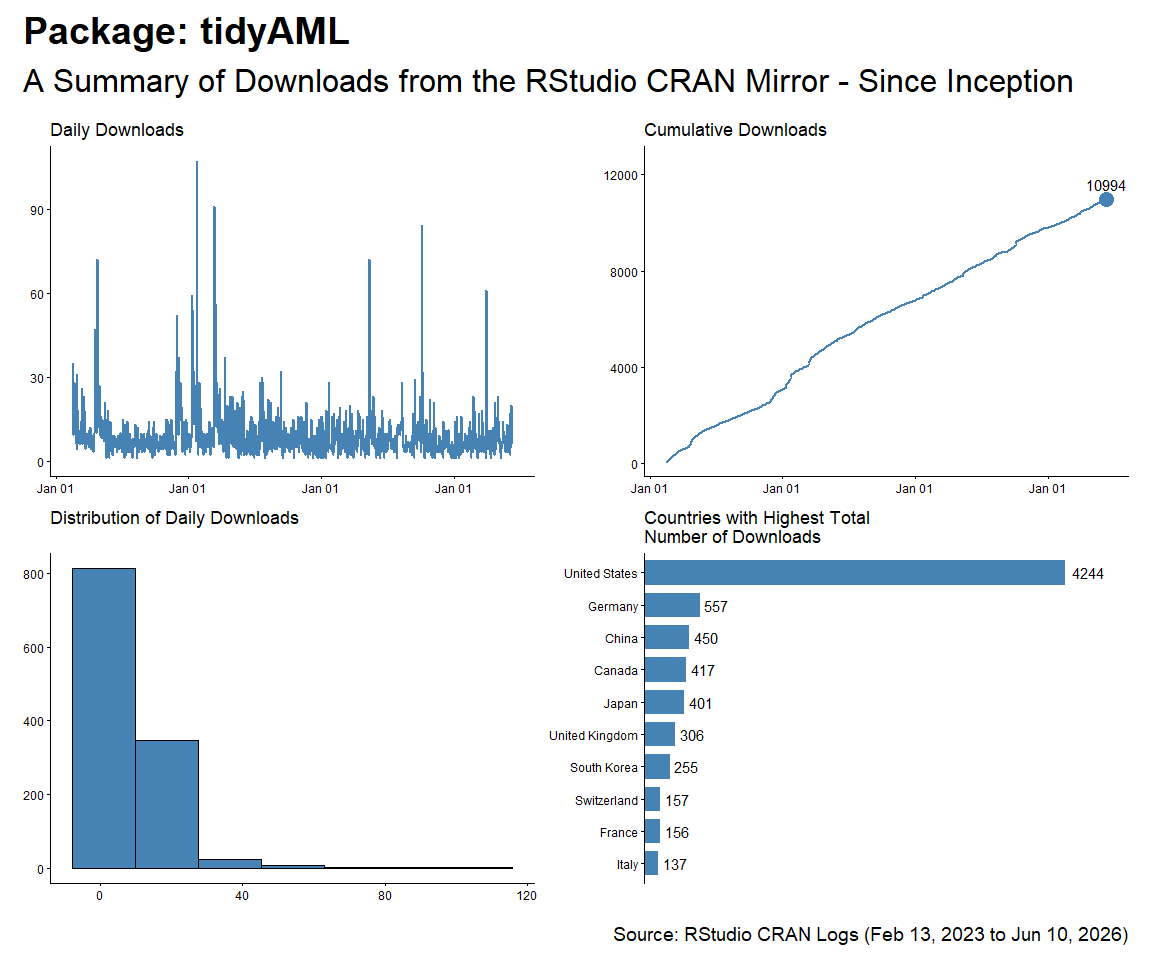# Table Data

``````total_downloads %>%
count(package, version) %>%
tidyr::pivot_wider(
id_cols       = version
, names_from  = package
, values_from = n
, values_fill = 0
) %>%
arrange(version) %>%
kable()
``````
version TidyDensity healthyR healthyR.ai healthyR.data healthyR.ts healthyverse tidyAML
0.0.1 1141 0 456 0 0 0 780
0.0.10 0 0 585 0 0 0 0
0.0.11 0 0 422 0 0 0 0
0.0.12 0 0 675 0 0 0 0
0.0.13 0 0 1825 0 0 0 0
0.0.2 0 0 1708 0 0 0 1785
0.0.3 0 0 469 0 0 0 156
0.0.4 0 0 563 0 0 0 0
0.0.5 0 0 1134 0 0 0 0
0.0.6 0 0 1478 0 0 0 0
0.0.7 0 0 804 0 0 0 0
0.0.8 0 0 931 0 0 0 0
0.0.9 0 0 708 0 0 0 0
0.1.0 0 348 0 0 577 0 0
0.1.1 0 1398 0 0 2079 0 0
0.1.2 0 1602 0 0 1090 0 0
0.1.3 0 422 0 0 1209 0 0
0.1.4 0 470 0 0 773 0 0
0.1.5 0 1120 0 0 614 0 0
0.1.6 0 2321 0 0 357 0 0
0.1.7 0 1119 0 0 1344 0 0
0.1.8 0 1479 0 0 1453 0 0
0.1.9 0 975 0 0 583 0 0
0.2.0 0 2214 0 0 586 0 0
0.2.1 0 2148 0 0 402 0 0
0.2.10 0 0 0 0 495 0 0
0.2.11 0 0 0 0 515 0 0
0.2.2 0 0 0 0 640 0 0
0.2.3 0 0 0 0 637 0 0
0.2.4 0 0 0 0 258 0 0
0.2.5 0 0 0 0 556 0 0
0.2.6 0 0 0 0 415 0 0
0.2.7 0 0 0 0 807 0 0
0.2.8 0 0 0 0 1182 0 0
0.2.9 0 0 0 0 691 0 0
0.3.0 0 0 0 0 293 0 0
1.0.0 505 0 0 2973 0 2437 0
1.0.1 1296 0 0 9342 0 2259 0
1.0.2 0 0 0 1337 0 3558 0
1.0.3 0 0 0 1671 0 443 0
1.0.4 0 0 0 0 0 1427 0
1.1.0 541 0 0 0 0 0 0
1.2.0 627 0 0 0 0 0 0
1.2.1 443 0 0 0 0 0 0
1.2.2 668 0 0 0 0 0 0
1.2.3 690 0 0 0 0 0 0
1.2.4 2431 0 0 0 0 0 0
1.2.5 1878 0 0 0 0 0 0
1.2.6 681 0 0 0 0 0 0
``````total_downloads %>%
count(package) %>%
tidyr::pivot_wider(
names_from = package,
values_from = n
) |>
kable()
``````
TidyDensity healthyR healthyR.ai healthyR.data healthyR.ts healthyverse tidyAML
10901 15616 11758 15323 17556 10124 2721

``````p1 <- plot_cumulative_downloads_pkg(total_downloads, pkg = "healthyR")

f <- function(date) format(date, "%b %d, %Y")
patchwork_theme <- theme_classic(base_size = 24) +
theme(
plot.title   = element_text(face = "bold"),
plot.caption = element_text(size = 14)
)
p1 + p2 + p3 + p4 + p5 + p6 + p7 +
plot_annotation(
title    = "healthyR packages are on the Rise",
subtitle = "A Summary of Downloads from the RStudio CRAN Mirror - Since Inception",
caption  = glue::glue("Source: RStudio CRAN Logs ({f(start_date)} to {f(end_date)})"),
theme    = patchwork_theme
)
``````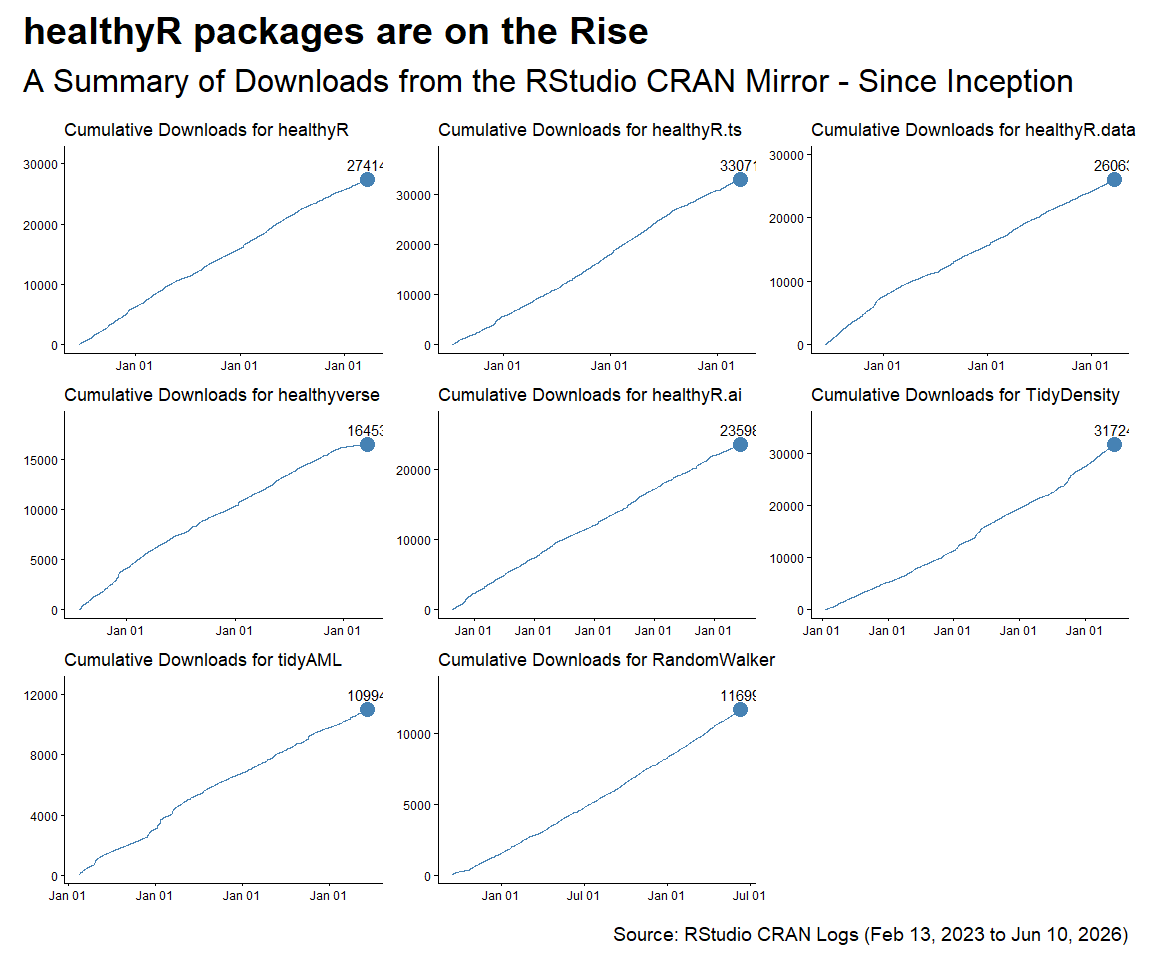# Thirty Day Run Post Release

``````pkg_rel <- readRDS("pkg_release_tbl.rds") |>
arrange(date) |>
group_by(package) |>
mutate(rel_no = row_number()) |>
ungroup()

lazy_dt() |>
select(date, package, version) |>
group_by(date, package, version) |>
summarise(dl_count = n()) |>
ungroup() |>
arrange(date) |>
group_by(package, version) |>
mutate(rec_no = row_number()) |>
mutate(cum_dl = cumsum(dl_count)) |>
filter(rec_no < 31) |>
ungroup() |>
mutate(pkg_ver = paste0(package, "-", version)) |>
collect()

release_tbl <- left_join(
x = thirty_day_runup_tbl,
y = pkg_rel
) |>
group_by(package) |>
fill(release_record, .direction = "down") |>
fill(rel_no, .direction = "down") |>
mutate(
release_record = as.factor(release_record),
rel_no = as.factor(rel_no)
) |>
ungroup()

latest_group_tbl <- release_tbl |>
group_by(package) |>
arrange(date, rec_no) |>
mutate(group_no = as.numeric(rel_no)) |>
filter(group_no == max(group_no)) |>
ungroup()

joined_tbl <- left_join(
x = thirty_day_runup_tbl,
y = latest_group_tbl
) |>
mutate(group_no = ifelse(is.na(group_no), FALSE, TRUE))

joined_tbl |>
ggplot(aes(x = rec_no, y = dl_count, group = as.factor(pkg_ver))) +
facet_wrap(~ package, scales = "free", ncol = 3) +
geom_line(aes(col = group_no)) +
scale_color_manual(values = c("FALSE" = "grey", "TRUE" = "red")) +
theme_minimal() +
labs(
x = "Day After Version Release",
col = "Latest Release"
)
``````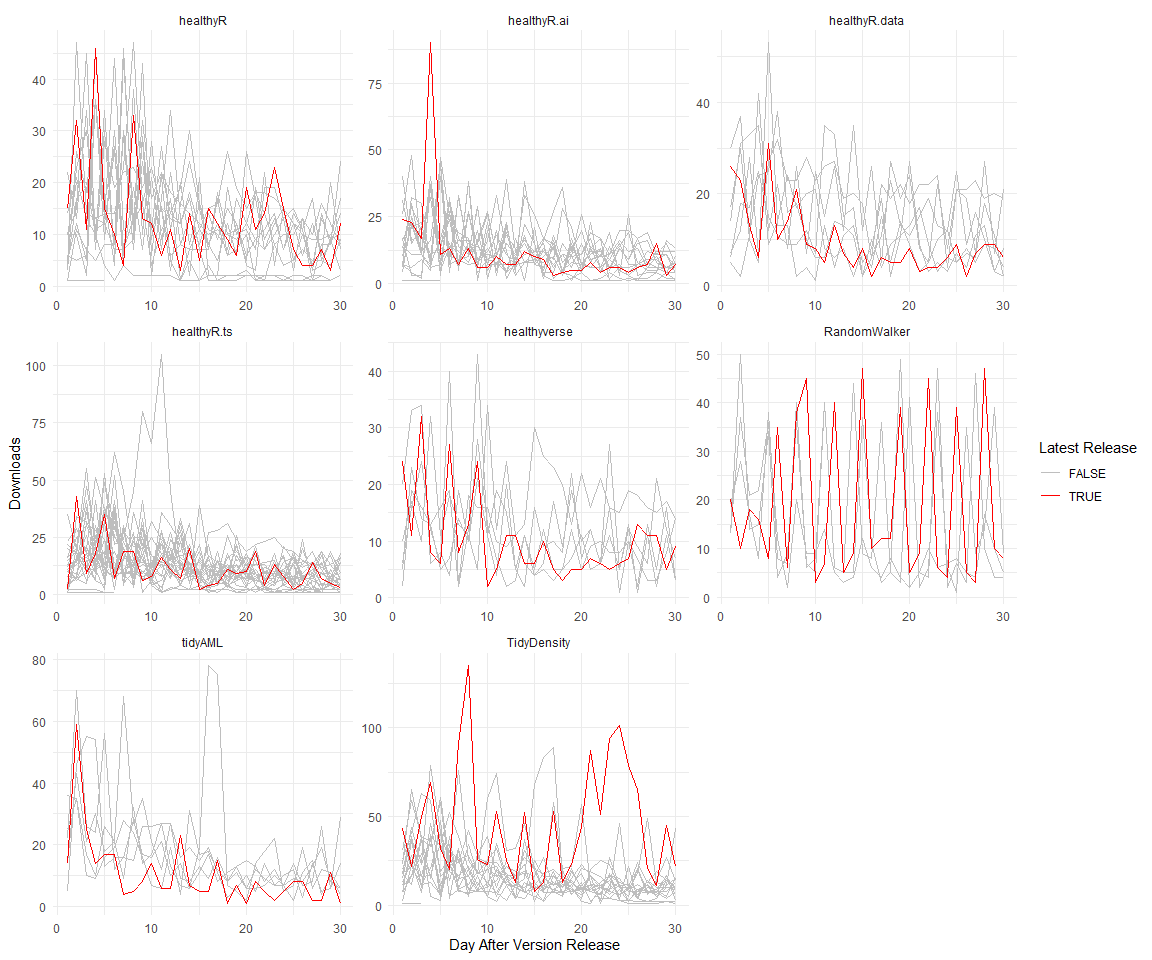``````joined_tbl |>
ggplot(aes(x = rec_no, y = cum_dl, group = as.factor(pkg_ver))) +
facet_wrap(~ package, scales = "free", ncol = 3) +
geom_line(aes(col = group_no)) +
scale_color_manual(values = c("FALSE" = "grey", "TRUE" = "red")) +
theme_minimal() +
labs(